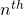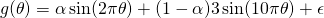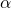[This article was first published on R – Daniel Oehm | Gradient Descending, and kindly contributed to R-bloggers]. (You can report issue about the content on this page here)
Want to share your content on R-bloggers? click here if you have a blog, or here if you don't.Generative Adversarial Networks are great for generating something from essentially nothing and there are some interesting uses for them. Most uses are some sort of image processing. Nvidia’s GauGAN is an impressive example, giving the user an MS paint-like interface and generating landscapes. You can give the beta a shot here.

I wanted to take a step back and use a small example to understand the basics and build some intuition behind GAN’s. There’s a tonne of information out there on how to fit a GAN to generate new hand drawn numbers, faces or Pokemon to varying success (the jury is still out as to whether or not the output can pass as Pokemon, but anyway). This isn’t the focus for this post. Instead, I’m going to simplify things further and use a GAN to generate sine waves.

## R + Python with {reticulate}

Keras and Tensorflow are used for this analysis. While there are R libraries I personally find it easier using Python via reticulate for deep learning tasks. You can find the code on Github. All the code bits in this post refer to functions from this repo.

For this to run correctly you’ll need Ananconda, Python 3.6-3.7 installed with keras and Tensorflow, as well as the standard libraries, numpy, pandas, etc. Along with python you’ll need reticulate installed and configured to use the appropriate version on Python. In short, run py_config() to initialise python for the session and py_available() to check if it’s all good to go. This can be tricky to set up and relies on how you’ve installed Python. If you have trouble refer to the reticulate cheat sheet and documentation.

## Example data

For training data I’m going to use two wave functions,with random noiseadded to throw in some minor variation.

get_training_data <- function(n = 200, m = 250, shape = list(a = c(1, 3), p = c(2, 10))) {
mat <- matrix(NA, nrow = n, ncol = m)
n_waves <- length(shape$a) for(k in 1:n){ ak <- shape$a[(k-1) %% n_waves + 1]
pk <- shape$p[(k-1) %% n_waves + 1] mat[k,] <- ak*sin(2*pi*seq(0, 1, length = m)*pk) + rnorm(m, 0, 0.05) } mat } train <- get_training_data() plot_waves(train) Nothing too complicated, just two distinct waves. Because we are generating training data using these two wave functions we only need to generate a handful of observations. ## Model There are two main components, the generator and discriminator. The generator generates new waves from random input, in this case a standard normal distribution. The discriminator sorts the real from the fake data. During training it will switch between training the discriminator and the generator. At each iteration both components perform better – the generator gets better at generating real observations and the discriminator gets better at determining whether or not the observation is real or fake. Like any neural network determining the number of hidden layers and sizes is more a process of experimentation than it is a science. For this example what I found worked well is, All are dense layers with LeakyReLU activation and 20% dropout. Given the input data is distinct it seems like overkill however I found this worked best. I’m sure other network configurations also work. The input data is time series data, in which case it is appropriate to use recurrent layers for the generator and discriminator. I actually found this to not be very successful. Either it takes far longer to train or just has trouble converging to a good solution, not saying it can’t be done though. For more challenging problems you’ll need more advanced models. Fortunately for this example we can keep it simple. ## Training To train the GAN on these two functions we simply run. gan(train, epochs = 2000) This will call the Python script with the GAN code, run it in Python for 2000 epochs and return the results. The training is saved in the global environment as x_train which is then able to be imported into the Python environment with r.x_train. A log file is created within the working directory and records the progress every 100 epochs. ## Output Once training has finished, view the output by py$gan_r$output. At eachiteration set by trace a set of waves are generated. The plot_waves function will plot a set from the final iteration. Recall, we only fed the GAN two sine functions, which makes the output below pretty cool. Here we see 12 randomly generated waves from the final iteration of the GAN. plot_waves(py$gan_r$output, seq(1, 24, 2)) With a single draw from a random normal distribution the GAN iteratively adjusted it’s weights until it learnt to generate and identify each wave. But also it learnt to generate new waves. What stands out here is the new waves appear to be some combination of the input waves. What we’ve done is found a really, really expensive and not particularly accurate way to estimate this…whereis between 0 and 1. This can be seen in the plot below where 12 waves have been plotted for different values of. Without explicitly telling the GAN what the functions were, it managed to learn them and explore the space between. While it estimated the frequency well it didn’t quite explore the whole range of amplitudes. They tend to range between 1.5-2.5 rather than 1-3. With more training it would probably get there. This took a few goes as training the GAN tends to converge to one of the input functions. By generating only one of the waves with high accuracy it can trick the discriminator into thinking it’s real every time. It’s a solution to the problem just not a very exciting one. With tuning we can get the desired result. Each starting value will correspond to some kind of wave. Out of the 12 random waves, 4 are very similar, right down to the two little spikes at the top of the second crest (see the waves in the third column). This suggests this wave is mapped to a set of values that may be drawn with a higher probability. ## Thoughts This isn’t as sexy as generating new landscape images using Paint but it’s helpful to understand what is going on within the guts of the GAN. It attempts to identify the key features in the observations making it distinct from random noise, pass as a real observation and map the space between. The same process is essentially happening at scale for more complex tasks. In this example it’s very inefficient to get the result we were looking for, but as the problem becomes more and more complex the trade-off makes sense. With an image example such as faces, the GAN will identify what a nose looks like and the range of noses that exist in the population, what an eye looks like and that they generally come in pairs at a slightly varying distance apart and so on. It will then generate eyes and noses along some space of possible eyes and noses, in the same way it generated a wave along some space of possible waves given the input. What’s interesting, the GAN only maps the space between. There are no examples where it generated a wave with a frequency greater than 10 or less than 2. Nor did it generate a wave with an amplitude greater than 3. The input waves essentially formed the boundaries of what could be considered real. There may be GAN variations that allow for this exploration. ## Code bits The easiest way to get the code for this example is from Github. Either clone or install. devtools::install_github("doehm/rgan") The Python component is quite long and don’t think there is much to gain pasting it here. As mentioned the trickiest part is going to be configuring Python, keras and tensorflow but the R bits should work. If you want to explore the output of the GAN the data is found at inst/extdata/gan-output.Rdata in the Github repo. This will show you how the GAN improved with each iteration. Each element of the list is a sample of generated waves at iteration 100, 200, …, 2000. This data is the basis of the output waves above. e.g. plot_waves(gan_output[], id = 1:12, nrow = 3) The code below created the title graphic. It is an area chart of waves using 48 values for. Perhaps worthy of an accidental aRt submission. shape <- list(a = c(1, 3), p = c(2, 10)) m <- 250 ln <- 48 pal <- c("#4E364B", "#8D4E80", "#D86C15", "#F3C925", "#48B2A8") map_dfr(seq(0, 1, length = ln), ~data.frame( x = seq(0, 1, length = m), y = .x*shape$a*sin(2*pi*seq(0, 1, length = m)*shape$p) + (1-.x)*shape$a*sin(2*pi*seq(0, 1, length = m)*shape\$p),
a = round(.x, 2))) %>% filter(x < 0.5) %>%
ggplot(aes(x = x, y = y, colour = a, fill = a, group = as.factor(a))) +
geom_area() +
theme_void() +
theme(legend.position = "none") +
scale_fill_gradientn(colors = colorRampPalette(pal)(200)) +
scale_colour_gradientn(colors = colorRampPalette(pal)(200))

The post Some basics and intuition behind GAN’s in R and Python appeared first on Daniel Oehm | Gradient Descending.

To leave a comment for the author, please follow the link and comment on their blog: R – Daniel Oehm | Gradient Descending.

R-bloggers.com offers daily e-mail updates about R news and tutorials about learning R and many other topics. Click here if you're looking to post or find an R/data-science job.
Want to share your content on R-bloggers? click here if you have a blog, or here if you don't.

# Never miss an update! Subscribe to R-bloggers to receive e-mails with the latest R posts.(You will not see this message again.)

Click here to close (This popup will not appear again)